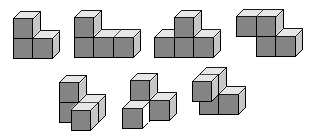## zondag 12 juni 2022

### The Soma Cube"The pieces of the Soma cube consist of all possible combinations of three or four unit cubes, joined at their faces, such that at least one inside corner is formed. There is one combination of three cubes that satisfies this condition, and six combinations of four cubes that satisfy this condition, of which two are mirror images of each other (see Chirality). Thus, 3 + (6 × 4) is 27, which is exactly the number of cells in a 3×3×3 cube."